Algebra 2 2-4 Independent Practice: More About Linear Equations
starstarstarstarstarstarstarstarstarstar
by Matthew Richardson
| 13 Questions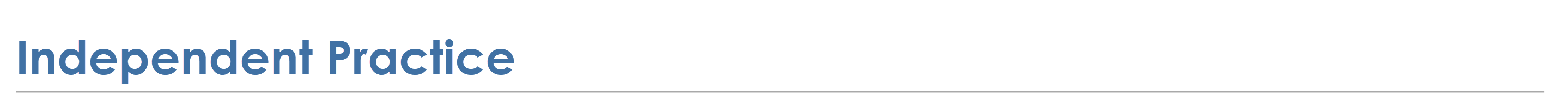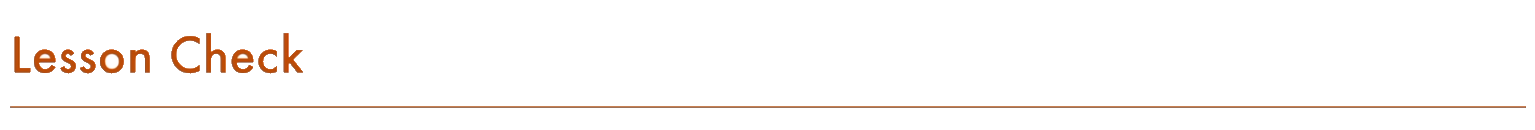1
1
10
A
B
C
D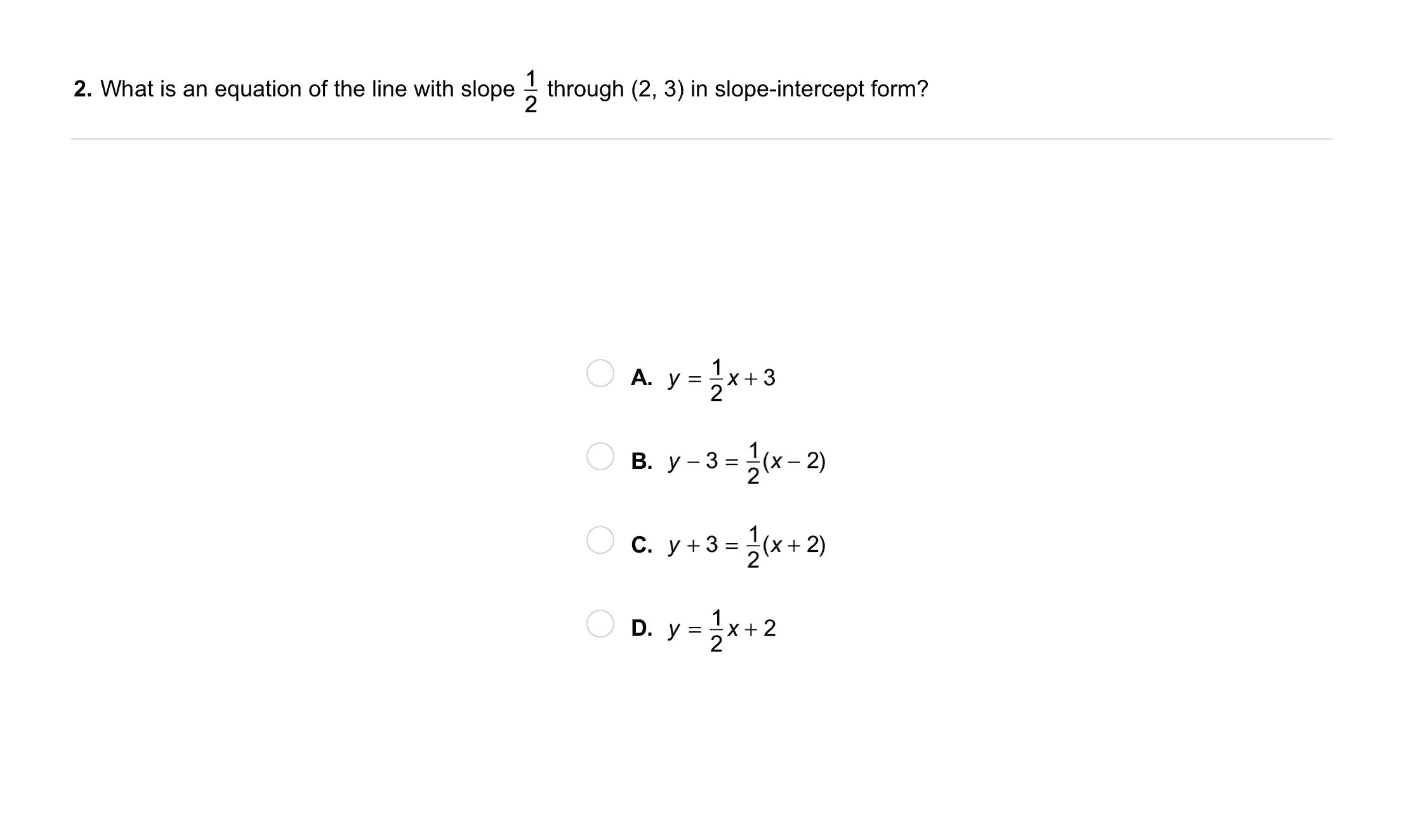2
2
10
A
B
C
D
3
30
Graphing: What are the intercepts of 3x + y = 6? Graph the equation. Be sure to include relevant graph detail: label axes, indicate units and scale on both axes, and use arrows to represent end behavior, as appropriate.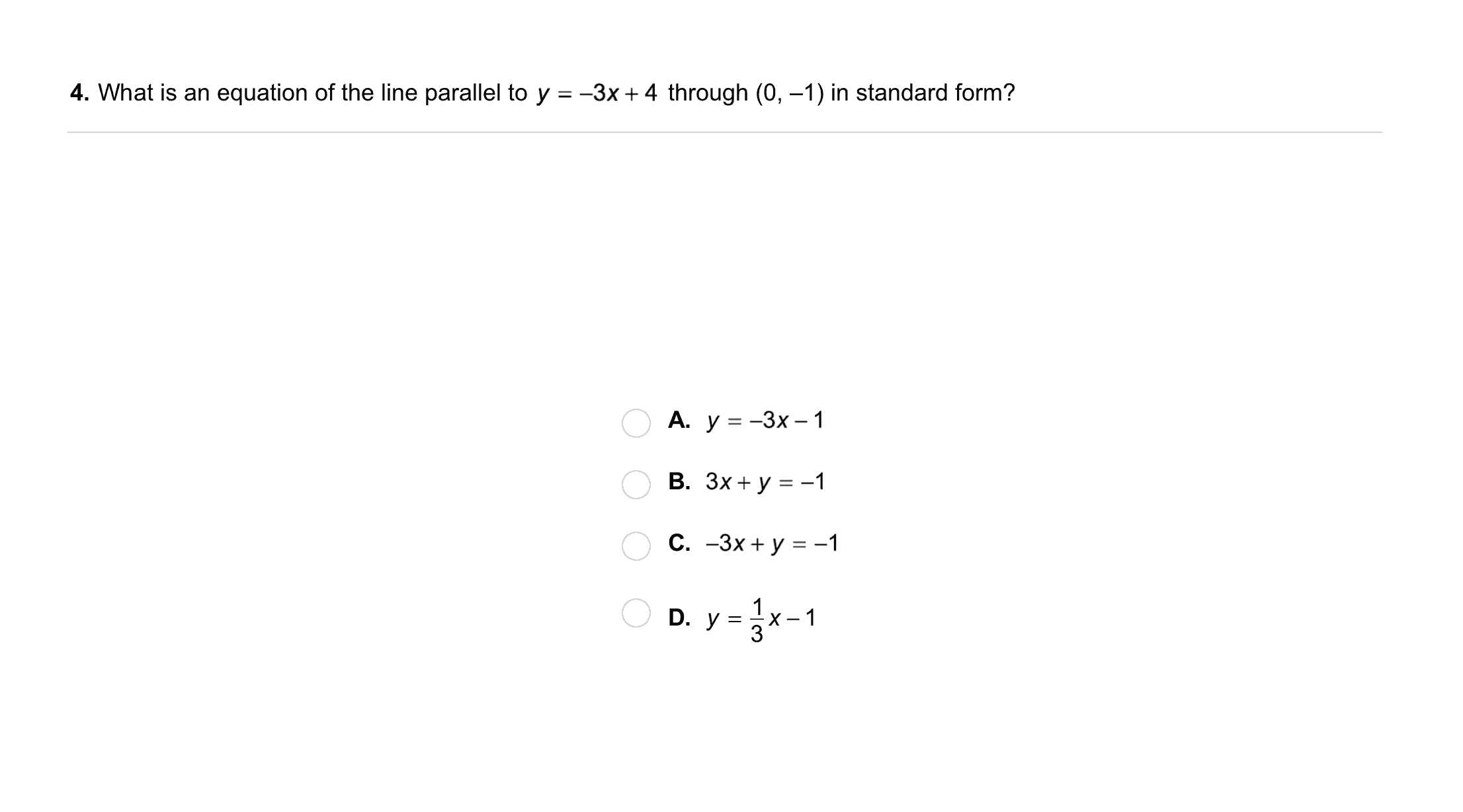4
4
10
A
B
C
D5
5
10
A
B
C
D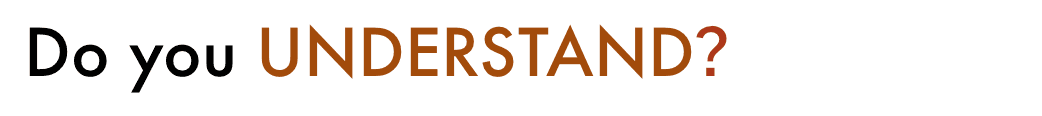6
10
Vocabulary: Tell whether the equation is in slope-intercept, point-slope, or standard form.
y + 2 = -2(x - 1)
slope-intercept form
standard form
point-slope form
7
10
Vocabulary: Tell whether the equation is in slope-intercept, point-slope, or standard form.
y = -¼x + 9
slope-intercept form
standard form
point-slope form
8
10
Vocabulary: Tell whether the equation is in slope-intercept, point-slope, or standard form.
-x - 2y = 1
slope-intercept form
standard form
point-slope form
9
10
Vocabulary: Tell whether the equation is in slope-intercept, point-slope, or standard form.
y - 3 = 4x
slope-intercept form
standard form
point-slope form
10
10
Understanding: Which form would you use to write the equation of a line if you knew its slope and x-intercept? Explain.
11
10
Understanding: If the intercepts of a line are (a, 0) and (0, b), what is the slope of the line? Assume that a and b are both greater than 0.

Enter only the slope, in simplified fraction form.
12
10
Error Analysis: Your friend says the line y = -2x + 3 is perpendicular to the line x + 2y = 8. Do you agree? Explain.
No; After converting the second equation to slope-intercept form, we can see that its slope is -1/2. Since -2 and -1/2 are not opposite reciprocals, the lines are not perpendicular.
Yes; After converting the second equation to slope-intercept form, we can see that its slope is 1/2. Since -2 and 1/2 are opposite reciprocals, the lines are perpendicular.
13
10
Reflection: Math Success